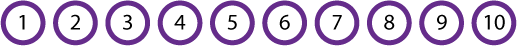# Maths

##Maths

At Front Lawn we develop children's mathematical thinking through a problem solving approach where skills and knowledge are mastered within a range of contexts. We pay particular attention to acquiring essential core skills as well as deepening understanding of concepts by giving our children a range of opportunities to analyse and talk about their mathematical thinking.

### Problem solving

We use the principles from Talk for Writing to provide opportunities for children to experience, explore and manipulate a range of mathematical concepts through guided, group and individual tasks. Key mathematical vocabulary is introduced, practised and used to explain mathematical thinking so that it becomes part of a child's everyday language.

Problems are not instantly calculable, often follow a line of enquiry and require children to use higher order reasoning skills. Children are expected to make links with prior learning and known strategies. They will consider different approaches to problem solving and establish efficient calculation methods.

### Fluency

It is essential for all our pupils to learn instant recall of mathematical facts. Known facts should include pairs of numbers up to 10, 20 and 100 and multiplication facts up to 12x12.The ability to instantly recall such number facts will help raise a child's confidence and ability to think creatively to solve mathematical problems.If you have ten counters numbered 1 to 10 , how many can you put into pairs that add to 8 ?
Can you use them all?

Thorough knowledge of number facts help in many calculations,

e.g. 47                            47

-14                          +14                                      Knowing that 4+3=7, 7-3=4 will make this calculation easy to complete

_____                      _____

_____                      _____

Our children will explore and analyse solutions to mathematical concepts to enable them to become fluent in the fundamentals of mathematical thinking. Through varied and frequent practice, and opportunities to discuss approaches, the children will develop the ability to recall and apply mathematical knowledge rapidly and accurately.

### Calculations

Children are taught written calculations in line with expectations from the National Maths Curriculum. We have high expectations to ensure age-appropriate methods are learned, evaluated and applied through a contextual approach.

4 1 1 7 4 Visitors
Top